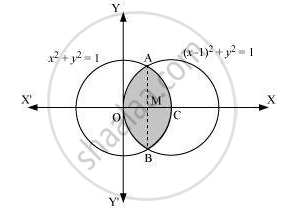# Find the Area of the Region Bounded by the Curves (X -1)2 + Y2 = 1 and X2 + Y2 = 1, Using Integration. - Mathematics

#### Question

Sum

Find the area of the region bounded by the curves (x -1)2 + y2 = 1 and x2 + y2 = 1, using integration.

#### Solution

The area bounded by the curves, (x – 1)2 + y2 = 1 and x2 + y 2 = 1, is represented by the shaded area asOn solving the equations, (x – 1)2 + y2 = 1 and x2 + y 2 = 1, we obtain the point of intersection as A(1/2, sqrt3/2) and B(1/2, -sqrt3/2)

It can be observed that the required area is symmetrical about x-axis.
∴ Area OBCAO = 2 × Area OCAO
We join AB, which intersects OC at M, such that AM is perpendicular to OC.
The coordinates of M are (1/2, 0).

⇒ Area OCAD = Area OMAO + Area MCAM

= [ int_0^(1/2) sqrt(1 - (x - 1)^2) dx + int_(1/2)^1 sqrt(1 - x^2 ) dx ]

= [ (x -1)/2 sqrt(1 - (x - 1)^2) + 1/2 sin^-1(x -1)]_0^(1/2) + [ x/2 sqrt(1 - x)^2 + 1/2 sin^-1 x]_(1/2)^1

= [ - 1/4 sqrt( 1 - (-1/2)^2) + 1/2 sin^-1(1/2 - 1) - 1/2 sin^-1 (-1)] + [1/2 sin^-1 - 1/4 sqrt(1 - (1/2)^2) - 1/2sin^-1 (1/2)]

= [- sqrt3/8 + 1/2(- pi/6) - 1/2 (- pi/2)] + [1/2(pi/2) - sqrt3/8 - 1/2(pi/6)]

= [-sqrt3/4 - pi/12 + pi/4 + pi/4 - pi/12 ]

= [-sqrt3/4 - pi/6 + pi/2]

= [2pi/6 - sqrt3/4]

Therefore, required area OBCAO = 2 xx (2pi/6 - sqrt3/4 ) = (2pi/3 - sqrt3/2) units

Is there an error in this question or solution?